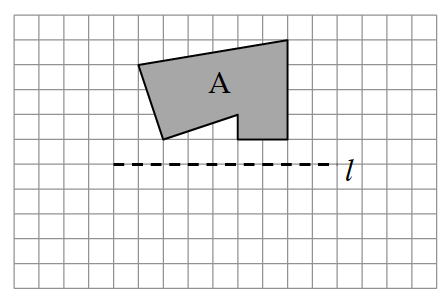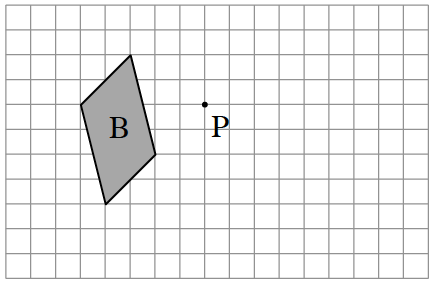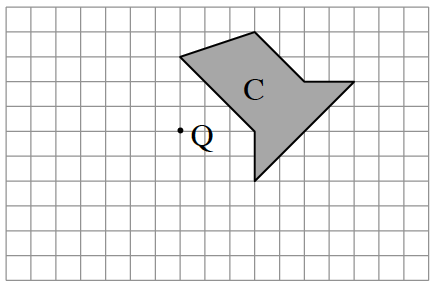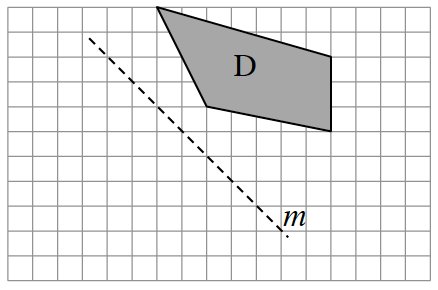### Home > CCG > Chapter 1 > Lesson 1.2.6 > Problem1-109

1-109.

Copy the diagrams below on graph paper. Then find each result when each indicated transformation is performed.

1. Reflect $A$ across line $l$.1. Rotate $B$ $90º$ counterclockwise ($\circlearrowleft$) about point $P$.1. Rotate $C$ $180º$ about point $Q$.1. Reflect $D$ across line $m$.Use the eTool below to view the diagrams and their answers. Be sure to hide both before going to the next figure.
Click the link at right for the full version of the eTool: 1-109 HW eTool